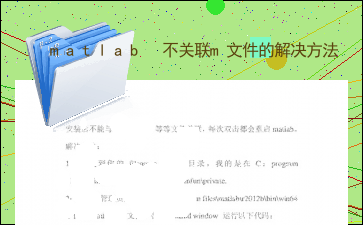• 以下为《matlab 不关联m文件的解决方法》的无排版文字预览，完整格式请下载下载前请仔细阅读文字预览以及下方图片预览。图片预览是什么样的，下载的文档就是什么样的。安装后能与.m，.mat,.fig等等文件关联，每次...

以下为《matlab 不关联m文件的解决方法》的无排版文字预览，完整格式请下载

下载前请仔细阅读文字预览以及下方图片预览。图片预览是什么样的，下载的文档就是什么样的。安装后不能与.m，.mat,.fig等等文件关联，每次双击都会重启matlab。解决办法：1：先找到你的fileassoc.m所在目录，我的是在C：program files\matlab\r2012b\toolbox\matlab\winfun\private.2：右键用管理员身份运行，C：program files\matlab\r2012b\bin\win64下面的matlab.exe文件。在command window 运行以下代码：

cwd=pwd;

cd([matlabroot '\toolbox\matlab\winfun\private']);

% fileassoc('add',{'.m','.mat','.fig','.p','.mdl',['.' mexext]}); % win7 64位试过，没效果

cd(cwd);

附上修改开始目录的办法：将matlab锁定在任务栏：把matlab.exe 拖到win7最下面的任务栏；

修改开始目录：右键matlab图标，再右键弹出来的matlab(r2012b)，属性进行设置就可以了。

[全文已结束，注意以上仅为全文的文字预览，不包含图片和表格以及排版]

以上为《matlab 不关联m文件的解决方法》的无排版文字预览，完整格式请下载

下载前请仔细阅读上面文字预览以及下方图片预览。图片预览是什么样的，下载的文档就是什么样的。

展开全文• 解决matlab不关联m文件的问题 步骤： 1.解压 2.将解压文件中的associateFiles.m添加到当前工作路径 3.命令行中输入associateFiles 4.回车，生成MatlabFileAssocFix.reg文件 5.运行这个reg文件 6.重启MATLAB
• 可通过添加fileassoc.m文件或注册表两种方法，关联各个版本的m文件，使用时直接点击就可以打开源程序。方便使用。
• 解决matlab不关联m文件的问题

千次阅读 2016-08-20 18:37:41
新安装matlab2016a时发现没有关联m文件，找了好多资料发现这个比较好用 1.下载文件：下载地址 2.解压 3.将解压文件中的associateFiles.m添加到当前工作路径 4.双击打开associateFiles.m文件 5.按...

新安装matlab2016a时发现没有关联m文件，找了好多资料发现这个比较好用

1.下载文件：下载地址

2.解压

3.将解压文件中的associateFiles.m添加到当前工作路径

4.双击打开associateFiles.m文件

5.按F5运行，生成MatlabFileAssocFix.reg文件

6.双击运行这个reg文件

展开全文matlab 关联m文件
• 解决matlab 2019 对m文件无法识别或无法拖拽打开的问题，笔者用的是matlab 2019b ，亲测有效
• 以管理员身份运行matlab打开matlab的help窗口，找到MATLAB / User's Guide / Desktop Tools and Development Environment / Startup and Shutdown / Starting and Quitting the MATLAB Program / Association Files ...

本文内容均摘抄自网络，备忘。

以管理员身份运行matlab

打开matlab的help窗口，找到MATLAB / User's Guide / Desktop Tools and Development Environment / Startup and Shutdown / Starting and Quitting the MATLAB Program / Association Files with MATLAB on Windows Platforms，往下翻直接找到下面这样的链接，直接点击，MATLAB会帮你自动关联。

Run utility to associate files with .fig extension with MATLAB Run utility to associate files with .m extension with MATLAB Run utility to associate files with .mat extension with MATLAB Run utility to associate files with .mdl extension with MATLAB Run utility to associate MATLAB with MEX-files Run utility to associate MATLAB with P-files Run utility to associate MATLAB with all of these file types: FIG, M, MAT, MDL, MEX, and P

最新版还是有些小改进的，还不错。不过就是有个问题一直存在，就是m文件的图标显示的很难看，一个缩小的matlab图标摆在那里，看起来很别扭，而且双击打开m文件时，不管matlab有没有打开，总是自动打开一个新的matlab，这怎么受得了。。

一般解决方法就是重建文件关联，试着在文件右键菜单里选择打开方式->选择默认程序，发现默认程序是Matlab Starter

Application，那双击打开肯定是新建matlab窗口了。将默认程序改为matlab安装目录下的bin文件夹里的matlab.exe程序，发现问题仍然存在。

网上搜了一下，有两个地方介绍了解决方法，我也稍作小结一下吧。

重装系统后，若没动MATLAB安装目录，则MATLAB不用重新安装。同样的原因，可以移动MATLAB安装目录。重装系统或移动matlab安装目录后，需要对MATLAB的相关文件重新建立关联。

方法有二：

1.使用代码

cwd=pwd;

cd([matlabroot

'\toolbox\matlab\winfun\private']);

mexext]}); %重点

cd(cwd);

disp('Changed Windows file associations. FIG, M,

MAT, MDL, MEX, and P files are now associated with MATLAB.')

2.使用matlab帮助

1.打开matlab，输入doc命令打开help

2.在help窗口中搜索Change Windows File

Associations3.找到Associating Files with MATLAB on Windows

Platforms(2011的帮助是这样，不同版本可能不一样)的对应解释

4.最后就是直接点击所需的文件关联，如下注意要使用管理员身份打开matlab，因为上面选中的文字说要修改注册表，这需要管理员权限。否则，会报错如下

Error using ==> fileassoc

No permissions to modify the

registry.

Win7下可以到matlab安装目录下的bin文件中，在matlab.exe文件上右击选择以管理员身份运行，就可以了。

点击最后一个建立所有的文件的关联，结果如下

Changed Windows file associations. FIG, M, MAT, MDL, MEX, and P files are now

associated with MATLAB.

其实上面两种方法是一样，将鼠标停到修改文件关联的链接上，状态栏会显示点击调用的代码，就是方法1中的代码。

如上面修改后，如果没有效果(图标不会立即改过来是正常的，因为上面的贴图有提到)，可以重启一下，这样在m文件的打开方式里就有了一个Matlab

(R2011a)的打开方式，这个才是真正要关联的打开方式。

另外，如果文件图标实在不能恢复的话，可以在我的电脑>工具>文件夹选项>文件类型中寻找各个文件名称修改。在键盘上按首字母可以快速搜索

图标文件在：D:\Program files\MATLAB\R2009b\bin\win32 中，后缀为ico。

而在Win7系统下，文件夹选项中取消了更改文件图标的功能。利用之前步骤将matlab文件与程序关联后，在控制面板>程序>默认程序>将文件类型或协议与程序关联中，找到任意一个matlab文件，如.m、.mat等，选中matlab程序，等待系统刷新一下，即可恢复图标。

本文引用地址：http://blog.sciencenet.cn/blog-515718-594234.html

上一篇：爱如风，如阳光

下一篇：Dynamical Systems Software

展开全文• （1）运行解压文件中的associateFiles.m （2）原文件夹下生成MatlabFileAssocFix.reg文件 （3）运行这个reg文件 （4）重启MATLAB
• 解决MATLAB2016b能与M文件关联的问题-附件资源
• MATLAB关联m文件Matlab编辑器-附件资源
• 第二步：启动安装的...第三步：将下载好的软件进行解压，将解压好的associateFiles.m文件直接拖到matlab的命令行中，然后matlab会出现run()的代码，回车运行这个代码，这时候在当前文件夹下会出现一个后缀为.reg...

第一步：首先下载这个文件链接：https://pan.baidu.com/s/1yy37wJjQlnn-_DKCXp4v6A ，提取码：84bn

第二步：启动安装的matlab文件

第三步：将下载好的软件进行解压，将解压好的associateFiles.m文件直接拖到matlab的命令行中，然后matlab会出现run()的代码，回车运行这个代码，这时候在当前文件夹下会出现一个后缀为.reg的文件，双击.reg文件。

第四步：关掉matlab，然后重新启动matlab，然后设置.m文件的默认打开方式即可。

展开全文Matlab
• step1：新建.m文件associateFiles.m，将以下代码写进该文件 function associateFiles(action, userExtList, fileStr) % associateFiles(action, extList, fileStr) % % Makes a registry files that can be used ...matlab
• 解决MATLAB2016b不能与M文件关联的问题

万次阅读 热门讨论 2018-01-20 23:09:35
MATLAB2016b能与M文件关联 最近安装MATLAB 2016b之后，发现能自动关联.m格式的文件。每次双击.文件都能找到MATLAB打开，只能先打开MATLAB软件，然后再找到m文件打开。太麻烦。。。参考网上的资料解决了这个...m文件 MATLAB
• 设置 vs2015 要在 VC 中成功编译 Matlab 引擎程序,必须包含引擎头文件 engine.h 并引入 Matlab 对应的 库文件 libmx.lib、libmat......Matlab 刷新屏幕 dsolve 符号计算解微分方程 Ee echo M 文件被执行指令的显示 ...
• 将associateFiles.m添加MATLAB工作路径，运行associateFiles.m 生成MatlabFileAssocFix.reg文件，软件外直接运行这个文件 重启MATLAB
• 解决现在MATLAB文件与软件无法关联的问题，可以实现MATLAB软件相关文件关联MATLAB
• Matlab2016a如何关联M文件

千次阅读 2018-11-11 22:44:41
前言 看似一个很简单的问题，...matlab2016a应该算是比较新的版本了，安装后发现MATLAB没有和M文件关联，那么每次打开M文件都需要重新打开一次MATLAB程序，很方便。下面就按照我的步骤解决这个小问题。 第一步...matlab
• 碰到matlab文件无法关联，尝试各种办法无效，最后使用这个.m档解决，具体使用说明，压缩说明里已经写好，请看压缩说明
• matlab2019与m文件关联

千次阅读 多人点赞 2019-05-21 13:02:33
找到网上很多用associateFiles的文件与m文件关联，但是对于matlab2019根本能用，现在提供新的associateFiles文件，亲测有效。 1.下载文件并把associateFiles放置于matlab文件夹下，在matlab里面运行该文件。 2....
• 下载文件，在MATLAB里面运行associateFiles.m这个文件 等待运行完毕，会生成：...运行完MatlabFileAssocFix.reg之后，找一个.m文件，再右键选择“打开方式”，就可以找到“MATLAB2018b”这个打开方式了。
• 如何将Matlab2019b和M文件关联

千次阅读 2020-02-16 20:45:25
Matlab关联M文件 Matlab是数学建模中一个常用的数据分析的软件，编写M脚本文件是对其进行处理的基本内容。对于刚刚安装的matlab软件，当你右键想要打开一个M文件，就会发现没有matlab的打开方式，这样每次都要先...
• .m文件与matlab的关联

千次阅读 2019-07-25 11:11:42
.m文件不显示默认图标，变为文本文件图标怎么办？ 遇到这种问题网上常见的两种解决方式就是： 删注册表，然后从新关联matlab starter，但是你会发现这样关联之后，并不是你想要的图标，而是和matlab.exe一模一样的...matlab
• MATLAB安装后经常出现m文件不关联matlab打开，很烦恼。网上有一些设置教程，比如： 链接一 按照链接方式一方式二设置后出现报错。 修改注册表亦没用。 下面链接 链接二 链接三 链接四 都没有效果 ...
• 之前的Matlab生成的.m文件无法自动关联Matlab 2018b。 当时的.m文件打开方式中没有MATLAB 2018b的选项。 在其他打开方式中选择了matlab，但是每次打开.m文件都会打开一个新的matlab程序，异常麻烦。 二，...MATLAB 配置
• 编写一个Windows注册表文件，该文件解决了Windows中MATLAB文件关联的问题，例如m文件未在MATLAB中打开或在新实例中打开。 也可以用于在不同安装版本的MATLAB之间切换文件关联。 该文件中包含详细的帮助。 已在...matlab
• 新安装MATLAB2016a之后，发现MATLAB没有和m文件关联 每次打开m文件后都会重新打开一次MATLAB主程序 后来发现这样的办法，亲测有效 下载压缩包： http://pan.baidu.com/s/1nuUf8UH 解压路径 将解压文件中的...MATLAB...

matlab不关联m文件matlab 订阅